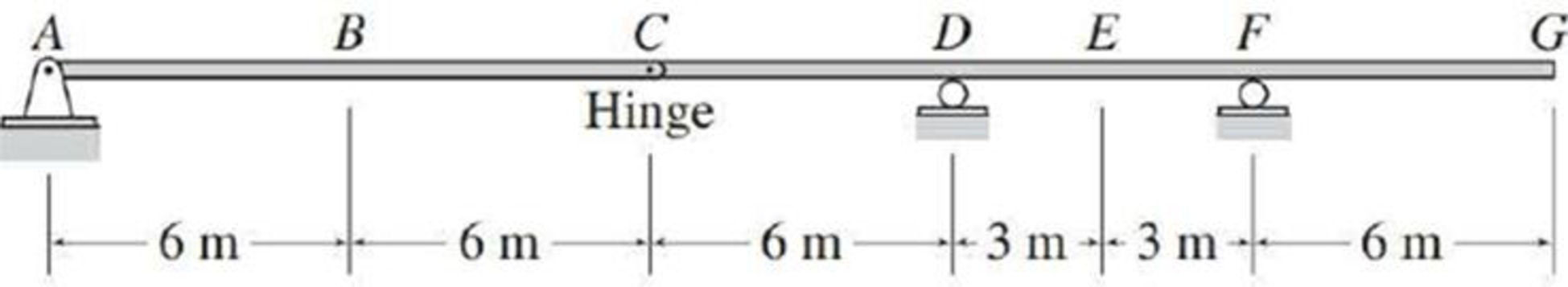# Draw the influence lines for the shear and bending moment at point B and the shear at internal hinge C of the beam shown in Fig. P8.20. FIG. P8.20, P8.21, P8.22

#### Solutions

Chapter
Section
Chapter 8, Problem 21P
Textbook Problem
147 views

## Draw the influence lines for the shear and bending moment at point B and the shear at internal hinge C of the beam shown in Fig. P8.20.FIG. P8.20, P8.21, P8.22

To determine

Draw the influence lines for the shear and bending moment at point B.

Draw the influence lines for the shear at internal hinge C.

### Explanation of Solution

Calculation:

Influence line for shear at point B:

Apply a 1 kN unit moving load at a distance of x from left end A.

Sketch the free body diagram of frame as shown in Figure 1.

Refer Figure 1.

Consider the unit load at a variable position x to the left hinge C. (placed portion AC of the beam 0x<12m).

Find the vertical support reaction (Ay) at A for portion AC.

Take Moment at hinge C from left end A.

Consider clockwise moment as positive and anticlockwise moment as negative.

ΣMCAC=0Ay(12)1(12x)=012Ay=12xAy=1x12

Consider the unit load at a variable position x to the right hinge C. (Place portion CG of the beam 12m<x30m).

Find the vertical support reaction (Ay) at A for portion CG.

Take Moment at hinge C from left end A.

Consider clockwise moment as positive and anticlockwise moment as negative.

ΣMCAC=0Ay(12)=0Ay=0

Thus, the equations of the influence line ordinate for Ay are,

Ay=1x12, 0x<12m (1)

Ay=0, 12m<x30m (2)

Refer Figure 1.

Find the equation of influence line ordinate for the vertical reaction (Dy) using equilibrium equation.

Apply moment equilibrium at F.

Consider clockwise moment as positive and anticlockwise moment as negative.

ΣMF=0Ay(24)+Dy(6)1(24x)=06Dy=24x24AyDy=4x64Ay (3)

Find the influence line ordinate of vertical reaction (Dy) for portion AC (0x<12m).

Substitute 1x12 for Ay in Equation (3).

Dy=4x64(1x12)=4x64+x3=x+2x6=x6

Find the influence line ordinate of vertical reaction (Dy) for portion CG(12m<x30m).

Substitute 0 for Ay in Equation (3).

Dy=4x64(0)=4x6

Thus, the equations of the influence line ordinate for Dy are,

Dy=x6, 0x<12m (4)

Dy=4x6, 12m<x30m (5)

Refer Figure 1.

Find the equation of influence line ordinate for the vertical reaction (Fy) using force equilibrium equation.

Consider the vertical forces equilibrium condition, take the upward force as positive (+) and downward force as negative ().

ΣFy=0Ay+Dy+Fy=1Fy=1AyDy (6)

Find the influence line ordinate of vertical reaction (Fy) for portion AC(0x<12m).

Substitute 1x12 for Ay and x6 for Dy in Equation (6).

Fy=1(1x12)(x6)=11+x12x6=x12

Find the influence line ordinate of vertical reaction (Fy) for portion AC(12m<x30m).

Substitute 0 for Ay and 4x6 for Dy in Equation (6).

Fy=1(0)(4x6)=14+x6=x63

Thus, the equations of the influence line ordinate for Fy are,

Fy=x12, 0x<12m (7)

Fy=x63, 12m<x30m (8)

Find the equation of shear at B of portion AB(0x<6m).

Sketch the free body diagram of the section AB as shown in Figure 2.

Refer Figure 2.

Apply equilibrium equation of forces.

Consider upward force as positive (+) and downward force as negative ().

ΣFy=0

Ay1SB=0SB=Ay1

Substitute 1x12 for Ay.

SB=(1x12)1=x12

Find the equation of shear at B of portion BG(6m<x30m).

Sketch the free body diagram of the section BG as shown in Figure 3.

Refer Figure 3.

Apply equilibrium equation of forces.

Consider upward force as positive (+) and downward force as negative ().

ΣFy=0

SB1+Dy+Fy=0SB=1DyFy (9)

Find the influence line ordinate of shear at B(SB) for portion BC (6m<x<12m).

Substitute x6 for Dy and x12 for Fy in Equation (9).

SB=1(x6)(x12)=1x6+x12=1x12

Find the influence line ordinate of shear at B(SB) for portion CG(12m<x30m).

Substitute 4x6 for Dy and x63 for Fy in Equation (9).

SB=1(4x6)(x63)=14+x6x6+3=3+0+3=0

Thus, the equations of the influence line ordinate for SB are,

SB=x12, 0x<6m (10)

SB=1x12, 6m<x<12m (11)

SB=0, 12m<x30m (12)

Find the influence line ordinate of SB using Equation (10), (11), and (12) and summarize the values in Table 1.

 x (m) Points Influence line ordinate of SB(kN/kN) 0 A 0 6 B− ‑0.5 6 B+ 0.5 12 C 0 18 D 0 21 E 0 24 F 0 30 G 0

Sketch the influence line diagram for the shear at pointB as shown in Figure 4.

Influence line for moment at point B:

Refer Figure 2.

Find the equation of bending moment at B of portion AB(0x<6m).

Take moment at B.

Consider clockwise moment as positive and anticlockwise moment as negative.

MB=Ay(6)1(6x)

Substitute 1x12 for Ay.

MB=(1x12)(6)1(6x)=6x2+6+x=x2

Refer Figure 3.

Find the equation of bending moment at B of portion BG(6m<x30m).

Take moment at B.

Consider clockwise moment as negative and anticlockwise moment as positive.

MB=1[24(30x)]+Dy(12)+Fy(18)=24+30x+12Dy+18Fy=6x+12Dy+18Fy (13)

Find the influence line ordinate of moment at B(MB) for portion BC(6m<x<12m)

### Still sussing out bartleby?

Check out a sample textbook solution.

See a sample solution

#### The Solution to Your Study Problems

Bartleby provides explanations to thousands of textbook problems written by our experts, many with advanced degrees!

Get Started

Find more solutions based on key concepts
Find the equation that best fits the following set of data points. Compare the actual and predicted y values.

Engineering Fundamentals: An Introduction to Engineering (MindTap Course List)

When might you choose to use a database instead of a spreadsheet? (170)

Enhanced Discovering Computers 2017 (Shelly Cashman Series) (MindTap Course List)

MDI stands for _________ ___________ ________.

Precision Machining Technology (MindTap Course List)

6. How would you level DFDs?

Systems Analysis and Design (Shelly Cashman Series) (MindTap Course List)

Find the resultant of the distributed load acting on the flat plate.

International Edition---engineering Mechanics: Statics, 4th Edition

What DBA activities support end users?

Database Systems: Design, Implementation, & Management

Who must be provided with safety data sheets (SDSs)?

Welding: Principles and Applications (MindTap Course List)

If your motherboard supports ECC DDR3 memory, can you substitute non-ECC DDR3 memory?

A+ Guide to Hardware (Standalone Book) (MindTap Course List)